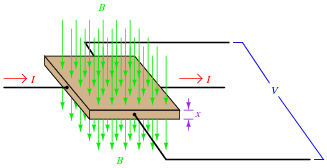# DC Transducers

## DC Electric Circuits

• #### Question 1

Research and describe what the Hall effect is, and list some practical uses of it.

• #### Question 2

The following equation is a general model for Hall effect devices, describing the voltage produced by a Hall element given the current through it (I), the magnetic flux density (B) passing through perpendicular to the current, and the thickness (x) of the Hall element. The variable K is a constant accounting for variations in material composition and temperature, and for any combination of units represented by the other variables:

 VHall = K IB xExplain what this equation means with regard to the effect of each variable (I, B, and x) on the Hall voltage generated. Identify whether each of the variables has a direct or an inverse effect on the output voltage.